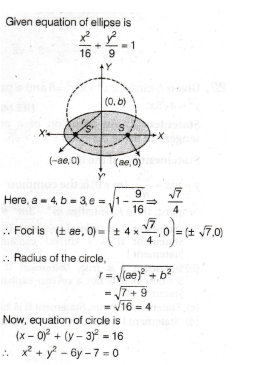# The equation of the circle passing through the foci of the ellipse x^2/16+y^/9=1 , and having centre at (0, 3) isSaurabh Koranglekar
2 years ago2 years ago

x^2/16+y^2/9=1

a^2=16 , b^2=9

b^2=a^2(1-e^2)

b^2=a^2-a^2.e^2

a^2.e^2=a^2-b^2=16–9=7

ae=+/-(7)^1/2

F(+/-ae,0) or (+/-7^1/2,0)

Eq. Of circle is:-

(x-x1)^2+(y-y1)^2=r^2

(x-0)^2+(y-3)^2=[(+/-7^1/2–0)^2+(0–3)^2]

x^2+y^2–6y+9=[7+9]

x^+y^2–6y+9–16=0

x^2+y^2–6y-7=0 ,